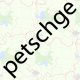# Given a lat/lon, how do I find the precise position on the tile?

 10 4 Given a latitude and longitude, I can find the correct tile number - but how do I find the correct position (in pixel) on the tile? This question is marked "community wiki". asked 07 Sep '10, 08:14petschge 8.3k●21●73●98 accept rate: 21% 2 related to the question about coordinates to pixels (17 Feb '11, 14:37) emj

 12 When you calulate the tile number according to the wiki you will actually get a floating point number. The integer part indicates which tile you are (or should be) looking at. The fractional part indicates the position within the tile. As a tile is 256 pixel wide, multiplying the fractional part with 256 will give you the pixel position from the top left. answered 07 Sep '10, 08:16petschge 8.3k●21●73●98 accept rate: 21%Gnonthgol ♦ 13.8k●16●103●198 Can you show a detailed example? for example we have lat : 36 lon: 6 we create square earth map with real scale in autocad how we can find the position x,y of the coordinates in the map? i don't see an example or a formula in the wiki (14 Oct '21, 06:27) Seghier
 1 If you just want to see the position on the map, you can use mlat and mlon to get a marker. answered 07 Sep '10, 13:39scai ♦ 33.2k●21●308●458 accept rate: 23% what you mean by mlat and mlon? where is the formula? (14 Oct '21, 06:29) Seghier
 0 I've written the following conversion functions to convert from Lat/Long coordinates to OSM pixel x,y coordinates for a given zoom level. ``````Friend Sub LatLongToPixelXYOSM(ByVal latitude As Double, ByVal longitude As Double, ByVal zoomLevel As Integer, ByRef pixelX As Integer, ByRef pixelY As Integer) Dim MinLatitude = -85.05112878 Dim MaxLatitude = 85.05112878 Dim MinLongitude = -180 Dim MaxLongitude = 180 Dim mapSize = Math.Pow(2, zoomLevel) * 256 latitude = Clip(latitude, MinLatitude, MaxLatitude) longitude = Clip(longitude, MinLongitude, MaxLongitude) Dim p As PointF = New Point() p.X = CSng((longitude + 180.0) / 360.0 * (1 << zoomLevel)) p.Y = CSng((1.0 - Math.Log(Math.Tan(latitude * Math.PI / 180.0) + 1.0 / Math.Cos(toRadians(latitude))) / Math.PI) / 2.0 * (1 << zoomLevel)) Dim tilex As Integer = CInt(Math.Truncate(p.X)) Dim tiley As Integer = CInt(Math.Truncate(p.Y)) pixelX = ClipByRange((tilex * 256) + ((p.X - tilex) * 256), mapSize - 1) pixelY = ClipByRange((tiley * 256) + ((p.Y - tiley) * 256), mapSize - 1) End Sub Friend Sub PixelXYToLatLongOSM(ByVal pixelX As Integer, ByVal pixelY As Integer, ByVal zoomLevel As Integer, ByRef latitude As Double, ByRef longitude As Double) Dim mapSize = Math.Pow(2, zoomLevel) * 256 Dim tileX As Integer = Math.Truncate(pixelX / 256) Dim tileY As Integer = Math.Truncate(pixelY / 256) Dim p As PointF = New Point() Dim n As Double = Math.PI - ((2.0 * Math.PI * (ClipByRange(pixelY, mapSize - 1) / 256)) / Math.Pow(2.0, zoomLevel)) longitude = CSng(((ClipByRange(pixelX, mapSize - 1) / 256) / Math.Pow(2.0, zoomLevel) * 360.0) - 180.0) latitude = CSng(180.0 / Math.PI * Math.Atan(Math.Sinh(n))) End Sub Private Function ClipByRange(ByVal n As Double, ByVal range As Double) Return n Mod range End Function Private Function Clip(ByVal n As Double, ByVal minValue As Double, ByVal maxValue As Double) Return Math.Min(Math.Max(n, minValue), maxValue) End Function `````` answered 14 Nov '11, 10:27johnmeilak 25●1 accept rate: 0%
 0 In the editor, press CTRL+SHIFT+L to display the Location Panel. answered 09 Jan, 11:19joper34 11●1●1●3 accept rate: 0%
 toggle preview community wiki:

By Email:

Markdown Basics

• *italic* or _italic_
• **bold** or __bold__
• image?![alt text](/path/img.jpg "title")
• numbered list: 1. Foo 2. Bar
• to add a line break simply add two spaces to where you would like the new line to be.
• basic HTML tags are also supported

Question tags:

×57
×53
×43
×20
×2

question asked: 07 Sep '10, 08:14

question was seen: 37,120 times

last updated: 09 Jan, 11:24